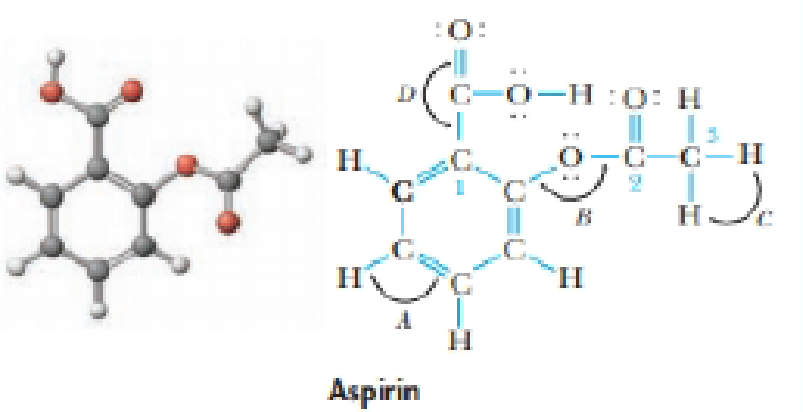Chapter 9, Problem 38GQ

Chapter
Section
Textbook Problem

The compound sketched below is acetylsalicylic acid, commonly known as aspirin.(a) What are the approximate values of the angles marked A, B, C, and D?(b) What hybrid orbitals are used by carbon atoms 1, 2, and 3ss?

(a)

Interpretation Introduction

Interpretation:

The approximate values of the angles marked A, B, C and D in the given molecule should be determined.

Concept Introduction:

VSEPR Theory:

As the name itself indicates that the basis for this theory is the electron pair that is bonded electron present in either single or double bonds or lone pair electrons, present in the valence shell tends to repel each other which then tend to be in position in order to minimize the repulsions.

Bond angle: The bond angle is the angle formed between three atoms at least two bonds.

TypeofmoleculeHybridaizationGeometryBondangleAX2spLinear180°AX3,AX2Bsp2Trigonalplanar120°AX4,AX3B,AX2B2sp3Tetrahedral109.5°AX5,AX4B,AX3B2,AX2B3sp3dTrigonalbipyramidal120°,90°AX6,AX5B,AX4B2sp3d2Octahedral90°ACentralatomXAtomsbondedtoABNonbondingelectronpairsonA

Explanation

Given structure of aspirin molecule is,

Value of the angles marked as A,B,C and D in the molecule can be determined as follows,

A is the bond angle between HCC bond. The central carbon atom is sp2 hybridized and so the bond angle value will be 120o

B is the bond angle between COC bond

(b)

Interpretation Introduction

Interpretation:

The hybrid orbitals which are used by the carbon atoms (indicated as 1,2, and 3) in the given aspirin molecule should be determined.

Concept Introduction:

Hybridization is the mixing of valence atomic orbitals to get equivalent hybridized orbitals that having similar characteristics and energy.

Geometry of a molecule can be predicted by knowing its hybridization.

sp3  hybrid orbitals is produced by hybridization of single s-orbital and three p-orbital.

sp2  hybrid orbitals is produced by hybridization between one s-orbital and two p-orbitals.

sp  hybrid orbitals is produced by hybridization of single s-orbital and single p-orbital.

Geometry of different types of molecule with respect to the hybridizations are mentioned are mentioned below,

TypeofmoleculeHybridaizationAtomicorbitalsusedforhybridaizationGeometryAX2sp1s+1pLinearAX3,AX2Bsp21s+2pTrigonalplanarAX4,AX3B,AX2B2sp31s+3pTetrahedralAX5,AX4B,AX3B2,AX2B3sp3d1s+3p+1dTrigonalbipyramidalAX6,AX5B,AX4B2sp3d21s+3p+2dOctahedralACentralatomXAtomsbondedtoABNonbondingelectronpairsonA

Geometry of different type of molecules with respect to the number of electron pairs are mentioned below,

TypeofMoleculeNo.ofatomsbondedtocentralatomsNo.oflonepairsoncentralatomArrangementofelectronpairsMolecularGeometryAB220LinearLinearAB330TrigonalplanarTrigonalplanarAB440TetrahedralTetrahedralAB550TrigonalbipyramidalTrigonalbipyramidalAB660OctahedralOctahedral

Still sussing out bartleby?

Check out a sample textbook solution.

See a sample solution

The Solution to Your Study Problems

Bartleby provides explanations to thousands of textbook problems written by our experts, many with advanced degrees!

Get Started